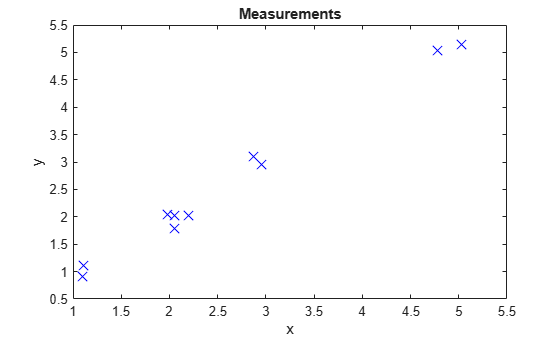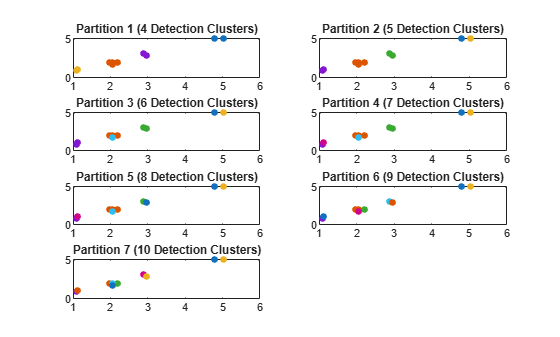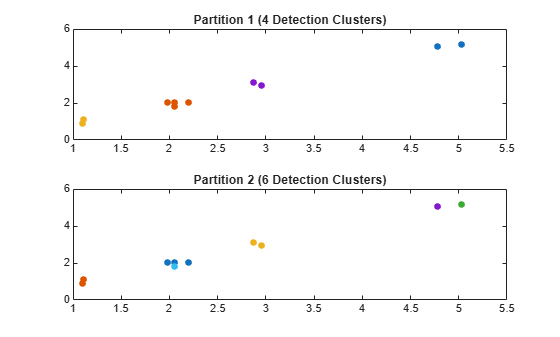# partitionDetections

Partition detections based on distance

Since R2019a

## Syntax

``partitions = partitionDetections(detections)``
``partitions = partitionDetections(detections,tLower,tUpper)``
``partitions = partitionDetections(detections,tLower,tUpper,'MaxNumPartitions',maxNumber)``
``partitions = partitionDetections(detections,allThresholds)``
``[partitions,indexDP] = partitionDetections(detections,allThresholds)``
``partitions = partitionDetections(detections,'Algorithm','DBSCAN')``
``partitions = partitionDetections(detections,epsilon,minNumPoints,'Algorithm','DBSCAN')``
``[partitions,indexDB] = partitionDetections(detections,epsilon,minNumPoints,'Algorithm','DBSCAN')``
``___ = partitionDetections(___,'Distance',distance)``

## Description

A partition of a set of detections is defined as a division of these detections into nonempty mutually exclusive detection cells. Using multiple distance thresholds, you can use the function to separate detections into different detection cells and get all the possible partitions using either distance-partitioning or density-based spatial clustering of applications with noise (DBSCAN). Additionally, you can choose the distance metric as Mahalanobis distance or Euclidean distance by specifying the `'Distance'` Name-Value pair argument.

### Distance Partitioning

Distance partitioning is the default partitioning algorithm of `partitionDetections`. In distance partitioning, a detection cluster comprises of detections whose distance to at least one other detection in the cluster is less than the distance threshold. In other words, two detections belong to the same detection cluster if their distance is less than the distance threshold. To use distance-partitioning, you can specify the `'Algorithm'` Name-Value argument as `'Distance-Partitioning'` or simply do not specify the `'Algorithm'` argument.

example

````partitions = partitionDetections(detections)` returns possible partitions for `detections` using the distance-partitioning algorithm. By default, the function uses the distance partitioning algorithm and considers all real value Mahalanobis distance thresholds between 0.5 and 6.25 and returns a maximum of 100 partitions. ```
````partitions = partitionDetections(detections,tLower,tUpper)` specifies the lower and upper bounds of the distance thresholds, `tLower` and `tUpper`.```
````partitions = partitionDetections(detections,tLower,tUpper,'MaxNumPartitions',maxNumber)` specifies the maximum number of allowed partitions, `maxNumber`.```
````partitions = partitionDetections(detections,allThresholds)` specifies the exact thresholds considered for partition.```
````[partitions,indexDP] = partitionDetections(detections,allThresholds)` additionally returns an index vector `indexDP` representing the correspondence between all thresholds and the resulting partitions.```

### DBSCAN Partitioning

To use the DBSCAN partitioning, specify the `'Algorithm'` argument as `'DBSCAN'`.

````partitions = partitionDetections(detections,'Algorithm','DBSCAN')` returns possible partitions of the detections by using DBSCAN partitioning and ten distance threshold (epsilon or neighbor search radius) values linearly spaced between 0.25 and 6.25. By default, each cluster must contain at least three points. ```

example

````partitions = partitionDetections(detections,epsilon,minNumPoints,'Algorithm','DBSCAN')` specifies the distance thresholds `epsilon` and the minimum number of points per cluster `minNumPoints` of the DBSCAN algorithm.```
````[partitions,indexDB] = partitionDetections(detections,epsilon,minNumPoints,'Algorithm','DBSCAN')` additionally returns an index vector `indexDB` representing the correspondence between the threshold values `epsilon` and the resulting partitions.```

### Specify Distance Metric

Using the `'Distance'` Name-Value argument, you can specify the distance metric used in the partitioning.

````___ = partitionDetections(___,'Distance',distance)` additionally specifies the distance metric as `'Mahalanobis'` or `'Euclidean'`. Use this syntax with any of the input or output arguments in previous syntaxes. ```

## Examples

collapse all

Generate 2-D detections using `objectDetection`.

```rng(2018); % For reproducible results detections = cell(10,1); for i = 1:numel(detections) id = randi([1 5]); detections{i} = objectDetection(0,[id;id] + 0.1*randn(2,1)); detections{i}.MeasurementNoise = 0.01*eye(2); end```

Extract and display generated position measurements.

```d = [detections{:}]; measurements = [d.Measurement]; figure() plot(measurements(1,:),measurements(2,:),'x','MarkerSize',10,'MarkerEdgeColor','b') title('Measurements') xlabel('x') ylabel('y')```Generate partitions from the detections using distance partitioning and count the number of partitions.

```partitions = partitionDetections(detections); numPartitions = size(partitions,2);```

Visualize the partitions. Each color represents a detection cluster.

```figure() for i = 1:numPartitions numCells = max(partitions(:,i)); subplot(4,ceil(numPartitions/4),i); for k = 1:numCells ids = partitions(:,i) == k; plot(measurements(1,ids),measurements(2,ids),'.','MarkerSize',15); hold on; end title(['Partition ',num2str(i),' (',num2str(k),' Detection Clusters)']); end```Generate 2-D detections using `objectDetection`.

```rng(2018); % For reproducible results detections = cell(10,1); for i = 1:numel(detections) id = randi([1 5]); detections{i} = objectDetection(0,[id;id] + 0.1*randn(2,1)); detections{i}.MeasurementNoise = 0.01*eye(2); end```

Extract and display generated position measurements.

```d = [detections{:}]; measurements = [d.Measurement]; figure() plot(measurements(1,:),measurements(2,:),'x','MarkerSize',10,'MarkerEdgeColor','b') title('Measurements') xlabel('x') ylabel('y')```Generate partitions from the detections using DBSCAN and count the number of partitions.

```[partitions,index] = partitionDetections(detections,[1.6;2],2,'Algorithm','DBSCAN'); numPartitions = size(partitions,2);```

Visualize the partitions. Each color represents a detection cluster.

```figure() for i = 1:numPartitions numCells = max(partitions(:,i)); subplot(2,ceil(numPartitions/2),i); for k = 1:numCells ids = partitions(:,i) == k; plot(measurements(1,ids),measurements(2,ids),'.','MarkerSize',15); hold on; end title(['Partition ',num2str(i),' (',num2str(k),' Detection Clusters)']); end```From the index values, the first partition corresponds to an epsilon value of `2` and the second partition corresponds to an epsilon value of `1.6`.

`index`
```index = 1x2 uint32 row vector 2 1 ```

## Input Arguments

collapse all

Object detections, specified as an N-element array of `objectDetection` objects, an N-element cell array of `objectDetection` objects, or an N-element array of structures whose field names are the same as the property names of the `objectDetection` object, where N is the number of detections. You can create `detections` directly, or you can obtain `detections` from the outputs of sensor objects, such as `fusionRadarSensor`, `irSensor`, and `sonarSensor`.

Data Types: `cell`

Distance metric for partitioning, specified as `'Mahalanobis'` or `'Euclidean'`.

Distance Partitioning

Lower bound of the distance thresholds, specified as a scalar. This argument sets the lower bound of the distance thresholds considered for distance partitioning.

Example: `0.05`

Data Types: `double`

Upper bound of the distance thresholds, specified as a scalar. This argument sets the upper bound of the distance thresholds considered for distance partitioning.

Example: `0.98`

Data Types: `double`

Maximum number of allowed partitions for distance partitioning, specified as a positive integer.

Example: `20`

Data Types: `double`

All thresholds for distance partitioning, specified as an M-element real-valued vector. The function calculates partitions based on each threshold value provided in `allThresholds`. Note that multiple thresholds can result in the same partition, and the function output `partitions`, returned as an N-by-Q matrix with QM, contains only unique partitions.

Example: `[0.1;0.2;0.35;0.4]`

Data Types: `double`

DBSCAN

All thresholds for DBSCAN, specified as an M-element real-valued element vector. The function calculates partitions based on each threshold value provided in `epsilon`. Note that multiple thresholds can result in the same partition, and the function output `partitions`, returned as an N-by-Q matrix with QM, contains only unique partitions.

Example: `[0.1;0.2;0.35;0.4]`

Data Types: `double`

Minimum number of points for each cluster in the partition, specified as a positive integer that applies to all epsilon values or as an M-element vector of positive integers, where M is the length of the `epsilon` vector.

Example: `20`

Data Types: `double`

## Output Arguments

collapse all

Partitions of detections, returned as an N-by-Q matrix. N is the number of detections and Q is the number of partitions. Each column of the matrix represents a valid partition. In each column, the value of the ith element represents the identity number of the cluster that the ith detection belongs to. For example, given a partition matrix P, if P(i,j) = k, then in partition j, detection i belongs to cluster k.

Index vector for distance partitioning, returned as an M-element vector of positive integers. Each element of the index vector is a partition index yielded by the corresponding threshold value in the `allThreshould` input argument. For example, if `indexDP(i) = k`, then `allThresholds(i)` corresponds to the partition specified by `partitions(:,k)`.

Data Types: `double`

Index vector for DBSCAN, returned as an M-element vector of positive integers. Each element of the index vector is a partition index yielded by the corresponding threshold value in the `epsilon` input argument. For example, if `indexDB(i)` is `k`, then `epsilon(i)` corresponds to the partition specified by `partitions(:,k)`.

Data Types: `double`

 Granstrom, Karl, Christian Lundquist, and Omut Orguner. “Extended Target Tracking Using a Gaussian-Mixture PHD Filter.” IEEE Transactions on Aerospace and Electronic Systems 48, no. 4 (October 2012): 3268–86. https://doi.org/10.1109/TAES.2012.6324703.

 Ester, Martin, Hans-Peter Kriegel, Jörg Sander, and Xiaowei Xu. “A Density-Based Algorithm for Discovering Clusters in Large Spatial Databases with Noise.” In Proceedings of the Second International Conference on Knowledge Discovery and Data Mining, 226–31. KDD’96. Portland, Oregon: AAAI Press, 1996.